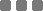## Wednesday, December 2, 2015

### Math: (Finite) Arithmetic Series Problem

I found this interesting problem, well someone asked me, and I answered that on a whiteboard. But because I ran out of marker, I type the steps here. So that it will last longer.
The problem goes like this (paraphrased):

The sum of first 10 members of an Arithmetic progression is 110.

The sum of 11th and 13th terms is -4.

Wut is the sum of the first and second members?

It looks "easy", maybe it can be done under 100 seconds. BUT, if we choose the "wrong path", we'll be super sweating.

Let's translate that to Math expressions

First, the denotational dilly

• Sum of n-finite series is usually denoted by \$ S_n \$.
• The \$ n^{th} \$ member (or term) is denoted by \$ a_n \$.
• The 1st term is denoted by \$ a \$ (or to be consistent, \$ a_1 \$).
Because I'm very not detergent, let's use just \$ a \$ instead.
• The difference between consecutive terms is denoted by \$ d \$ (as in difference).

Second, the finite Arithmetic formulas

These are the um, thing we probably forgot or not. As a reminder, they look like this:

• Arithmetic series (sum):

\$ S_n = n/2(2a + (n-1)d) \$

• The progression member/term:

\$ a_n = a + (n-1)d \$

If you don't believe me, try either one by substi...

Third, the concept

We need to expand the known variables using the formulas above, so then we'll generate a system of linear equations in two variables.

Let's then translate that

• \$ S_10 = 110 \$
• \$ a_11 + a_13 = -4 \$
• The question: \$ a_1 + a_2 = ? \$

➤ we need to find the \$ a \$ and \$ d \$, so then:

Expand the \$ S_10 = 110 \$

\$ S_10 = 110 \$

\$ 10/2(2a + (10 - 1)d) = 110 \$

\$ 5(2a + 9d) = 110 \$

\$ 2a + 9d = 110/5 \$

\$ 2a + 9d = 22 ... (1) \$ Our first linear equation

Expand the \$ a_11 + a_13 = -4 \$

\$ a_11 + a_13 = -4 \$

\$ (a + (11 - 1)d) + (a + (13 - 1)d) = -4 \$

\$ (a + 10d) + (a + 12d) = -4 \$

\$ 2a + 22d = -4 ...(2)\$ Second linear equation

Now we have 2 equations in 2 variables; we can find the value of each thing using basic elimination

\$ 2a + 9d = 22 ... (1) \$

\$ 2a + 22d = -4 ... (2)\$

------------------------

\$ -13d = 26 \$

\$ d = -2 \$

And then the \$ a \$; using substitution; let's take the 1st equation

\$ 2a + 9d = 22...(1)\$

\$ 2a + 9(-2) = 22 \$

\$ 2a + -18 = 22 \$

\$ 2a = 22 + 18 \$

\$ a = 40/2 \$

\$ a = 20 \$ COMPLETE!

The question is \$ a_1 + a_2 = ?\$

Expand that into:

\$ ⇔ (a + (1 - 1)d) + (a + (2 - 1)d)\$

\$ ⇔ (a) + (a + d)\$

\$ ⇔ 2a + d \$

Substitute \$ a = 20 \$ and \$ d = -2 \$

\$ ⇔ 2(20) + (-2) \$

\$ ⇔ 38 \$

WOOO!The other series/progression is called Geometric. Instead of (static) difference, it has (static) ratio between consecutive members.

There are also sequences with difference or ratio "stages", sort of mutating but with finite steps. Those cannot use the formulas above.

Also, there are infinite series/progressions.

Hm.Math: (Finite) Arithmetic Series Problem
https://monkeyraptor.johanpaul.net/2015/12/math-arithmetic-series.html